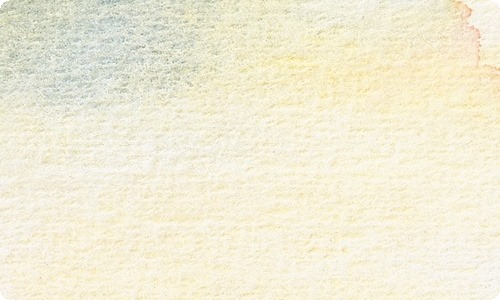﻿ 数一数教案-星极写作网

# 数一数教案1.学习运用图谱记忆歌词的方法，教幼儿初步学会唱歌曲，理解歌词内容，注意唱准虎、鹿、兔、狐、鼠的发音。

2.在学会演唱歌曲的基础上，用歌声表现出歌曲的情绪，尝试改变图片顺序进行重组演唱。

3.在学唱绕口令歌曲的过程中。感受绕口令的趣味性。

1.听音乐《秋天多么美》做小跑步进活动室。

2.出示图片，以谈话的方式引出课题。

3.发声练习

1=C 2/4

1 2 3 4| 5 - | 5 5 5 |5 5 5 | 5 4 3 2| 1 - ||

1.放音乐让幼儿完整欣赏歌曲，然后回答问题：

（1）歌曲叫什么名字？

（2）是几拍子 ……此处隐藏13303个字……，2个3，3个3，4个3，5个3 3+3+3+3+3=15

3．6+6+6+6=24

4．4+4+4+4+4+4=24

1．刚才我们数了熊猫和圆片，下面我们来数一数方格。

2．根据学生汇报板书

3．从刚才这些算式中你发现了什么？

2．汇报思考过程及数的方法和算式

3．组内交流

⑴列出的都是相同加数的加法算式

⑵不同数法可以列出不同的加法算式

实践应用：　　1．谈话：秋天到了，苹果成熟了。看这里有许多又红又大的苹果，一共多少个呢》？列出加法算式

2．5盘苹果是5个3相加，那么6盘，10盘，15盘呢？你能列式吗？

3．求50盘苹果有多少，你会算吗？能列式吗？

4．谈话：这时候用乘法会比较简单1．3+3+3+3+3+=15

2．3+3+3+3+3+3

3+3+3+3+3+3+3+3+3+3=？

3+3+3+3+3+3+3+3+3+3+3+3+3+3+3=？

3．3+…………………………+3=？

5+5+5=15（个） 10+10+10=30

3+3+3+3+3=15（个） 3+3+3+3+3+3+3+3+3+3=30

…… ……

6+6+6+6=24（个） 3+3+3+3+3=15

4+4+4+4+4+4=24（个） ……《数一数教案.doc》